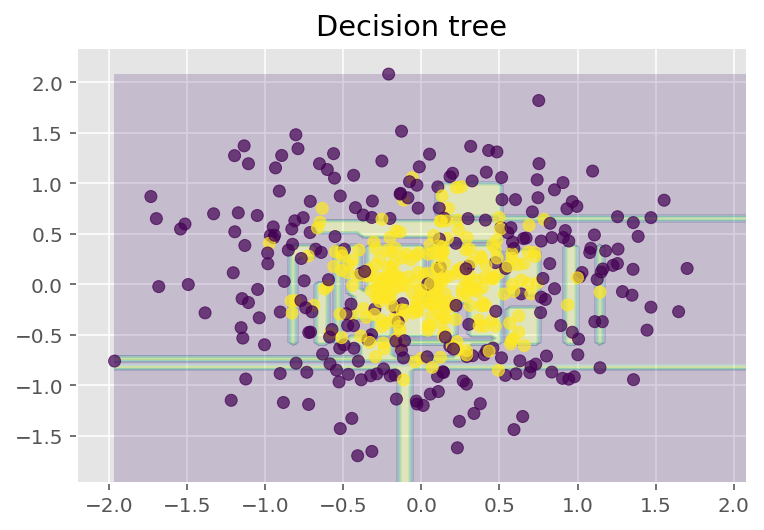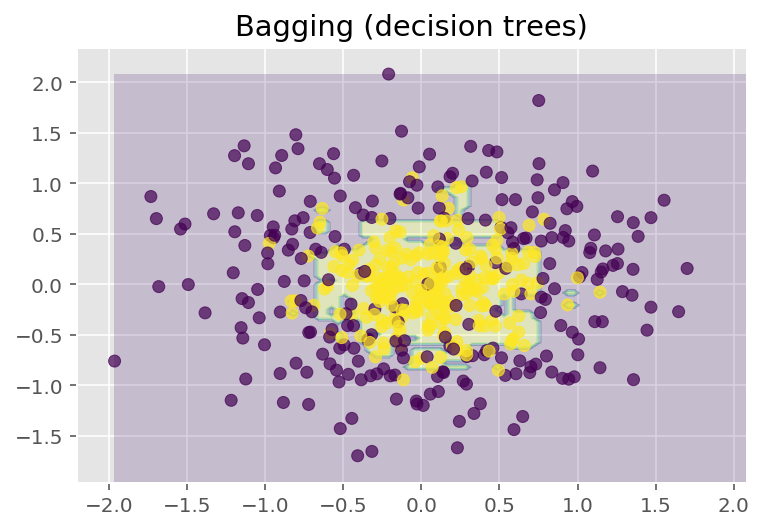## mlcourse.ai – Open Machine Learning Course¶

Authors: Vitaliy Radchenko, and Yury Kashnitsky. Translated and edited by Christina Butsko, Egor Polusmak, Anastasia Manokhina, Anna Shirshova, and Yuanyuan Pao. This material is subject to the terms and conditions of the Creative Commons CC BY-NC-SA 4.0 license. Free use is permitted for any non-commercial purpose.

This is a static version of a Jupyter notebook. You can also check out the latest version in the course repository, the corresponding interactive web-based Kaggle Notebook or a video lecture.

# Topic 5. Ensembles and random forest¶

## Article outline¶

$\DeclareMathOperator{\Var}{Var}$ $\DeclareMathOperator{\Cov}{Cov}$ $\DeclareMathOperator{\Corr}{Corr}$ $\DeclareMathOperator{\Err}{Err}$ $\DeclareMathOperator{\Bias}{Bias}$ $\DeclareMathOperator{\E}{\mathbb{E} }$

Leo Breiman managed to apply bootstrapping not only in statistics but also in machine learning. He, along with Adel Cutler, extended and improved the random forest algorithm proposed by Tin Kam Ho. They combined the construction of uncorrelated trees using CART, bagging, and the random subspace method.

Decision trees are a good choice for the base classifier in bagging because they are quite sophisticated and can achieve zero classification error on any sample. The random subspace method reduces the correlation between the trees and thus prevents overfitting. With bagging, the base algorithms are trained on different random subsets of the original feature set.

The following algorithm constructs an ensemble of models using the random subspace method:

1. Let the number of instances be equal to $\large \ell$, and the number of features be equal to $\large d$.
2. Choose $\large L$ as the number of individual models in the ensemble.
3. For each model $\large l$, choose the number of features $\large dl < d$. As a rule, the same value of $\large dl$ is used for all the models.
4. For each model $\large l$, create a training set by selecting $\large dl$ features at random from the whole set of $\large d$ features.
5. Train each model.
6. Apply the resulting ensemble model to a new instance by combining the results from all the models in $\large L$. You can use either majority voting or aggregation of the posterior probabilities.

## 1. Algorithm¶

The algorithm for constructing a random forest of $\large N$ trees goes as follows:

• For each $\large k = 1, \dots, N$:

• Generate a bootstrap sample $\large X_k$.
• Build a decision tree $\large b_k$ on the sample $\large X_k$:

• Pick the best feature according to the given criteria. Split the sample by this feature to create a new tree level. Repeat this procedure until the sample is exhausted.
• Building the tree until any of its leaves contains no more than $\large n_\text{min}$ instances or until a certain depth is reached.
• For each split, we first randomly pick $\large m$ features from the $\large d$ original ones and then search for the next best split only among the subset.

The final classifier is defined by: $$\large a(x) = \frac{1}{N}\sum_{k = 1}^N b_k(x)$$

We use the majority voting for classification and the mean for regression.

For classification problems, it is advisable to set $\large m = \sqrt{d}$. For regression problems, we usually take $\large m = \frac{d}{3}$, where $\large d$ is the number of features. It is recommended to build each tree until all of its leaves contain only $\large n_\text{min} = 1$ examples for classification and $\large n_\text{min} = 5$ examples for regression.

You can see random forest as bagging of decision trees with the modification of selecting a random subset of features at each split.

## 2. Comparison with Decision Trees and Bagging¶

In :
# Disable warnings in Anaconda
import warnings

import numpy as np

warnings.filterwarnings('ignore')

%matplotlib inline
from matplotlib import pyplot as plt

plt.style.use('ggplot')
plt.rcParams['figure.figsize'] = 10, 6
%config InlineBackend.figure_format = 'retina'

import seaborn as sns
from sklearn.datasets import make_circles
from sklearn.ensemble import (BaggingClassifier, BaggingRegressor,
RandomForestClassifier, RandomForestRegressor)
from sklearn.model_selection import train_test_split
from sklearn.tree import DecisionTreeClassifier, DecisionTreeRegressor

In :
n_train = 150
n_test = 1000
noise = 0.1

# Generate data
def f(x):
x = x.ravel()
return np.exp(-x ** 2) + 1.5 * np.exp(-(x - 2) ** 2)

def generate(n_samples, noise):
X = np.random.rand(n_samples) * 10 - 5
X = np.sort(X).ravel()
y = np.exp(-X ** 2) + 1.5 * np.exp(-(X - 2) ** 2)\
+ np.random.normal(0.0, noise, n_samples)
X = X.reshape((n_samples, 1))

return X, y

X_train, y_train = generate(n_samples=n_train, noise=noise)
X_test, y_test = generate(n_samples=n_test, noise=noise)

# One decision tree regressor
dtree = DecisionTreeRegressor().fit(X_train, y_train)
d_predict = dtree.predict(X_test)

plt.figure(figsize=(10, 6))
plt.plot(X_test, f(X_test), "b")
plt.scatter(X_train, y_train, c="b", s=20)
plt.plot(X_test, d_predict, "g", lw=2)
plt.xlim([-5, 5])
plt.title("Decision tree, MSE = %.2f"
% np.sum((y_test - d_predict) ** 2))

# Bagging with a decision tree regressor
bdt = BaggingRegressor(DecisionTreeRegressor()).fit(X_train, y_train)
bdt_predict = bdt.predict(X_test)

plt.figure(figsize=(10, 6))
plt.plot(X_test, f(X_test), "b")
plt.scatter(X_train, y_train, c="b", s=20)
plt.plot(X_test, bdt_predict, "y", lw=2)
plt.xlim([-5, 5])
plt.title("Bagging for decision trees, MSE = %.2f" % np.sum((y_test - bdt_predict) ** 2));

# Random Forest
rf = RandomForestRegressor(n_estimators=10).fit(X_train, y_train)
rf_predict = rf.predict(X_test)

plt.figure(figsize=(10, 6))
plt.plot(X_test, f(X_test), "b")
plt.scatter(X_train, y_train, c="b", s=20)
plt.plot(X_test, rf_predict, "r", lw=2)
plt.xlim([-5, 5])
plt.title("Random forest, MSE = %.2f" % np.sum((y_test - rf_predict) ** 2));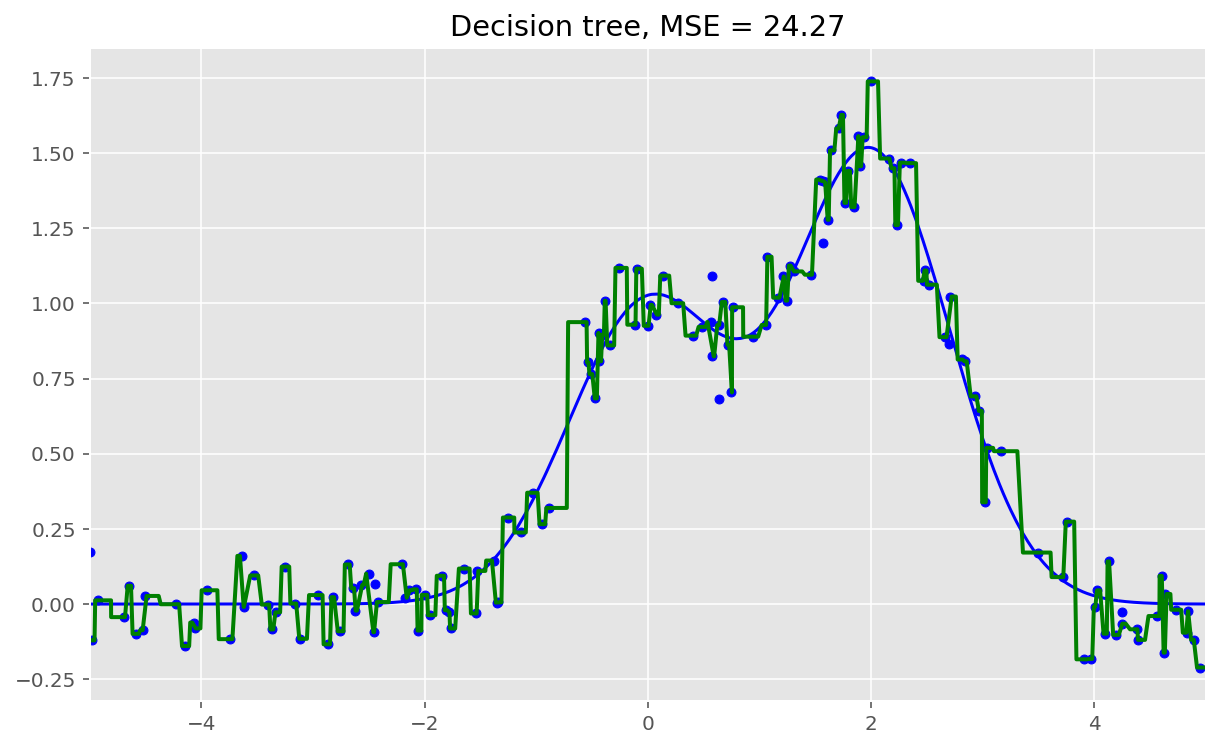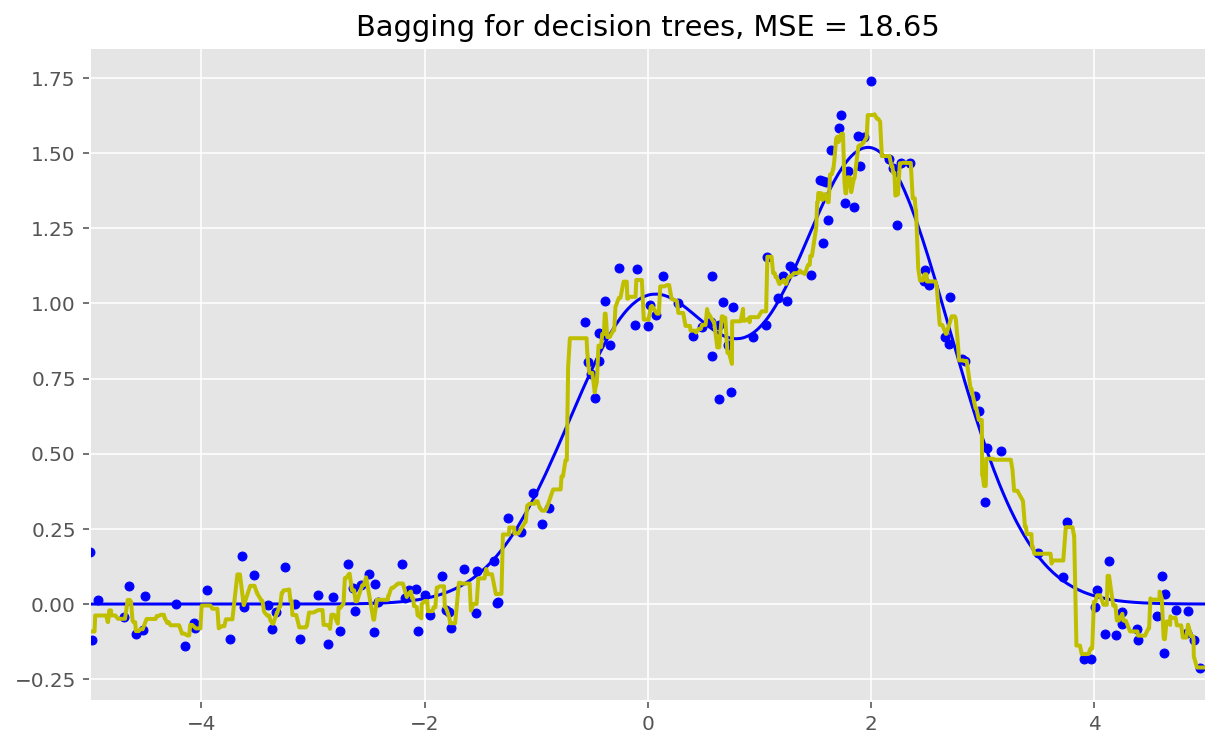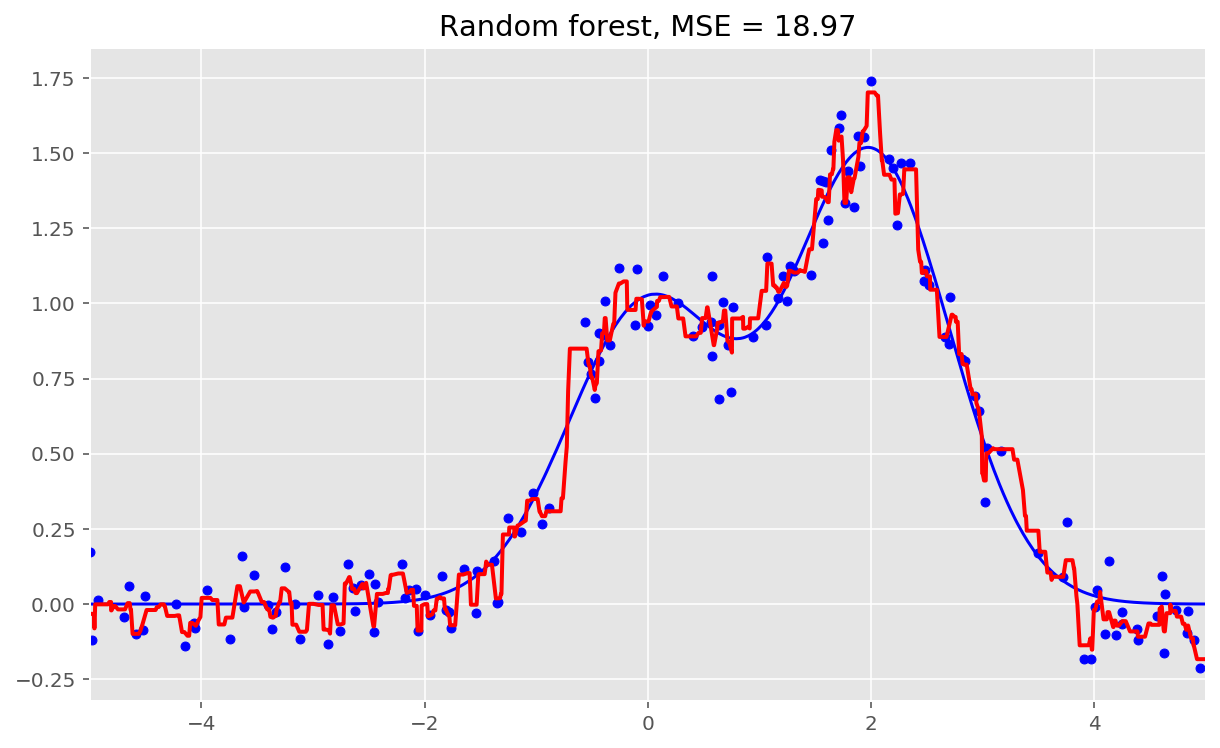As we can see from our graphs and the MSE values above, a random forest of 10 trees achieves a better result than a single decision tree and is comparable to bagging with 10 trees. The main difference between random forests and bagging is that, in a random forest, the best feature for a split is selected from a random subset of the available features while, in bagging, all features are considered for the next best split.

We can also look at the advantages of random forests and bagging in classification problems:

In :
np.random.seed(42)
X, y = make_circles(n_samples=500, factor=0.1, noise=0.35, random_state=42)
X_train_circles, X_test_circles, y_train_circles, y_test_circles = \
train_test_split(X, y, test_size=0.2)

dtree = DecisionTreeClassifier(random_state=42)
dtree.fit(X_train_circles, y_train_circles)

x_range = np.linspace(X.min(), X.max(), 100)
xx1, xx2 = np.meshgrid(x_range, x_range)
y_hat = dtree.predict(np.c_[xx1.ravel(), xx2.ravel()])
y_hat = y_hat.reshape(xx1.shape)
plt.contourf(xx1, xx2, y_hat, alpha=0.2)
plt.scatter(X[:,0], X[:,1], c=y, cmap='viridis', alpha=.7)
plt.title("Decision tree")
plt.show()

b_dtree = BaggingClassifier(DecisionTreeClassifier(),
n_estimators=300, random_state=42)
b_dtree.fit(X_train_circles, y_train_circles)

x_range = np.linspace(X.min(), X.max(), 100)
xx1, xx2 = np.meshgrid(x_range, x_range)
y_hat = b_dtree.predict(np.c_[xx1.ravel(), xx2.ravel()])
y_hat = y_hat.reshape(xx1.shape)
plt.contourf(xx1, xx2, y_hat, alpha=0.2)
plt.scatter(X[:,0], X[:,1], c=y, cmap='viridis', alpha=.7)
plt.title("Bagging (decision trees)")
plt.show()

rf = RandomForestClassifier(n_estimators=300, random_state=42)
rf.fit(X_train_circles, y_train_circles)

x_range = np.linspace(X.min(), X.max(), 100)
xx1, xx2 = np.meshgrid(x_range, x_range)
y_hat = rf.predict(np.c_[xx1.ravel(), xx2.ravel()])
y_hat = y_hat.reshape(xx1.shape)
plt.contourf(xx1, xx2, y_hat, alpha=0.2)
plt.scatter(X[:,0], X[:,1], c=y, cmap='viridis', alpha=.7)
plt.title("Random forest")
plt.show()